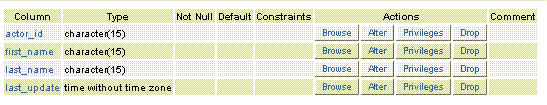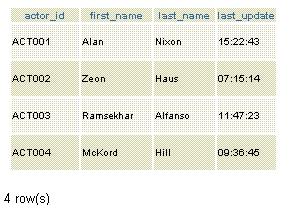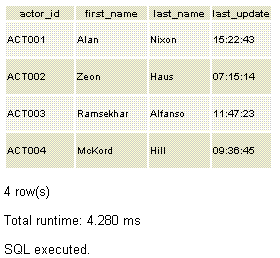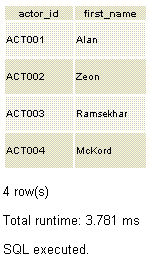# PostgreSQL SELECT

## SELECT Statement

PostgreSQL SELECT statement retrieves data from a single table or multiple tables or views (a virtual table).

In this document, we will discuss syntax, examples, and PostgreSQL with PHP code example of a SELECT statement.

Syntax:

SELECT select_list FROM table_expression [sort_specification]

Parameters

Parameter Description
select_list If * then denotes all columns of the table, else a list of the columns of the table or make calculations using the columns.
table_expression A single table, a combination of tables, JOINs(combination of records from two or more table) and subqueries (a query which a subset of another query). You may not use table_expression and use the SELECT command as a calculator instead.
sort_specification ORDER BY, LIMIT, and/or OFFSET clauses.

We will now create a table, insert some data into that table and then run SELECT statements.

Create Table

Code:

`````` CREATE table actor( actor_id character(15), first_name character(15), last_name character(15), last_update time without time zone)
```
```

Table StructureInsert data

Code:

``````INSERT INTO actor values ('ACT001','Alan','Nixon','15:22:43')INSERT INTO actor values ('ACT002','Zeon','Haus','07:15:14')INSERT INTO actor values ('ACT003','Ramsekhar','Alfanso','11:47:23')INSERT INTO actor values ('ACT004','McKord','Hill','09:36:45')
```
```

Status of the table after inserting dataPostgreSQL SELECT example1

If we want to fetch all rows from the actor table the following PostgreSQL SELECT statement can be used. Here in the statement below an asterisk(*) have used to fetch all rows.

SQL

Code:

``````SELECT * FROM actor
```
```

Output:PHP with PostgreSQL SELECT example 1

Code:

``````<!DOCTYPE html>
<html lang="en">
<meta charset="utf-8">
<title>PostgreSQL SELECT Example 1</title>
<meta name="description" content="If we want to fetch all rows from the actor table the following PostgreSQL SELECT statement can be used.">
<body>
<h1>List of all actors in the table</h1>
<?php
\$result = pg_query(\$db,"SELECT * FROM actor");
echo "<table>";
while(\$row=pg_fetch_assoc(\$result)){echo "<tr>";
echo "<td align='center' width='200'>" . \$row['actor_id'] . "</td>";
echo "<td align='center' width='200'>" . \$row['first_name'] . "</td>";
echo "<td align='center' width='200'>" . \$row['last_name'] . "</td>";
echo "<td align='center' width='200'>" . \$row['last_update'] . "</td>";
echo "</tr>";}echo "</table>";?>
</div>
</body>
</html>
```
```

PostgreSQL SELECT example2

If we want to fetch all rows from the columns actor_id and first_name columns from the actor table the following PostgreSQL SELECT statement can be used. Here in the statement below, we mention the specific columns.

SQL

Code:

``````SELECT actor_id, first_name  FROM actor
```
```

Output:PHP with PostgreSQL SELECT example 2

Code:

``````<!DOCTYPE html>
<html lang="en">
<meta charset="utf-8">
<title>PostreSQL SELECT Example 2</title>
<meta name="description" content="If we want to fetch all rows from the columns actor_id and first_name columns from the actor table the following PostgreSQL SELECT statement can be used.">
<body>
<h1>List of all actors in the table</h1>
<?php
\$result = pg_query(\$db,"SELECT actor_id, first_name FROM actor");
echo "<table>";while(\$row=pg_fetch_assoc(\$result)){echo "<tr>";
echo "<td align='center' width='200'>" . \$row['actor_id'] . "</td>";
echo "<td align='center' width='200'>" . \$row['first_name'] . "</td>";
echo "<td align='center' width='100'>" . \$row['last_name'] . "</td>";
echo "<td align='center' width='100'>" . \$row['last_update'] . "</td>";
echo "</tr>";}
echo "</table>";?>
</div>
</body>
</html>
```
```

Previous: ALTER TABLE
Next: WHERE Clause

﻿